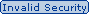#### Which is the equation used to derive a 64 Kbps bit rate?Send Via Email (Need Reachable Email ID)## 99.9% OFF All In One Supper Package

Which is the equation used to derive a 64 Kbps bit rate?
A. 2 x 8 kHz x 4-bit code words
B. 8 kHz x 8-bit code words
C. 2 x 4-bit code words x 8 kHz
D. 2 x 4 kHz x 8-bit code words